### IMO Shortlist 1982 problem 3

Kvaliteta:
Avg: 0.0
Težina:
Avg: 0.0
Consider infinite sequences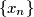$\{x_n\}$ of positive reals such that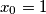$x_0=1$ and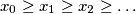$x_0\ge x_1\ge x_2\ge\ldots$.

a) Prove that for every such sequence there is an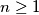$n\ge1$ such that: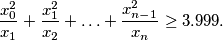b) Find such a sequence such that for all$n$: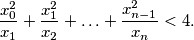Source: Međunarodna matematička olimpijada, shortlist 1982